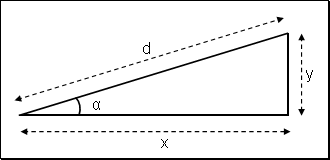Slope

For an ascent (or descent) route, the average slope Pt is the ratio between the gradient y and the projection x of the route distance d so : Pt = y / xFor a calculation with a low angle, x can be replaced by d, otherwise a precise calculation must be done as follow.

 Gradient (y): m (+ ascent, - descent) Distance (d): m
 Angle: ° Slope: %

Exemples of sites giving gradient and distance:
• OpenRunner
• ClimbByBike

Design of the calculator

Converter